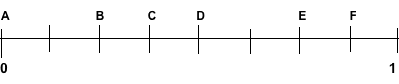Fraction Name
Compare Fractions
Vocabulary
Fractions on a
number line
100Write a fraction for the model

5/2

2 1/2

100

Complete the number sentence...< > =

1/8      6/8

<

Less than

100

Which number is the numerator?

¾

3

100What fraction is B?

B = 2/8

200

Write the fraction for the model.15/5

3

200

Complete the number sentence

7/10       3/10

>

greater than

200

Which number is the denominator?

1/2

2

2003/6

300

Write the fraction for the model.8/4

2

300

Complete the number sentence

10/12      10/12

=

equal to

300

What type of fractions are these:

6/5              11/3        9/4

improper fractions

300What fraction is A?

A = 2/6

400

Draw a model for this mixed number

2 3/4400

Complete the number sentence

1/2        1/3

greater than

400

What are these called?

2 1/2              3 1/8             6 2/3

Mixed Numbers

400

What fraction is A?3/4

500

Draw a model for this fraction:

6/5500

Complete the number sentence

1/8                     3/4

<

less than

500

When the numerator is larger than the denominator it is called...

Improper Fraction

5003/4

6/8

Click to zoom